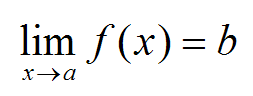# Limits made easy and continuity

Hi,
yesterday I wrote some basics about functions and today I will write about limits again, and when they are defined and when they are not.

Just to remind you, we will use limits on functions. Those functions will usually be plotted on graph so it is easier to work with them. Our first function we work with will be the one below:If you have not seen that yet, filled dot means that the point is inside of the function and circle means that the point is not there. Now when we speak of limit and if it is defined we have to get the same results as we approach from both sides.

What do I mean by this? Limit is basicly just approaching some number very very close. Take for example x=1. At that point in the function above, y equals 5. If we wanted to take the limit it would go as follow:

limx→1   f(x)                           

Now if we think about it before we get the result, we know that you can approach one thing in 1st dimension in two directions. Those are in limits noted as from the negative side (-) and positive side (+).

For the limit I wrote, (limx→1   f(x)) from both sides we will get the normal result as if we wanted to know what the function outputs but sometimes we just can not use the limit because  it does not exist.

Such an example would be for the following limit (still working with the first function):

limx→2   f(x)

In this limit we are trying to approach the number two but from the graph we can see that it is strange at that point. The function jumps so we should check the limit from both sides.

limx→2+   f(x) = 1
limx→2   f(x) = 2

Since the limits do not equal each other, the one before does not exist! We can have so called “one-sided limits” but they are not of much use as far as I know. Now we can actually create amazing definition for continous function!

Continous function is such that does not have any jumps or spaces in it.The function on the left is discontinous. This is because on one point there is space, which means that function is not defined there.. this is problem if you want to be continous.Another function is discontinous even that it is defined on all Xs. The problem lies in what I told you about, if we took the limitx→3 f(x) = undefined
This is because from positive and negative side we will get different result.Again the function is not continous. This time the  limit at 2 exists but it does not equal the value of the function.

limitx→2 f(x) ≠ f(2)This one on is not continous either. This is because if we approach from negative values we will get -∞ but if we approach from positive values we will get +∞. The limits do not equal so the function can not be continous.

From these points we can summarize what function has to have to be continous at one point.

1. Point there is defined, it is not empty circle!
2. Limit on the point exists.
3. The limit equals to the point on the function.

Or also:

1. f(a) is defined
2. limx→ a f(x) exists
3. limx→ a f(x) = f(a)

This is all from me for now, thanks to “analyzemath.com“. I did not need to create all the graphs again thanks to them.

Dragallur

Sadly wordpress is not able to create some normal indexing so I will write it like this. Normally limit is noted as this:Proper notation for limit where x approaching something is in the bottom.